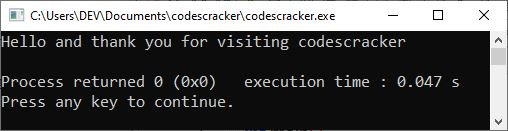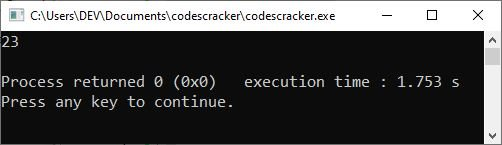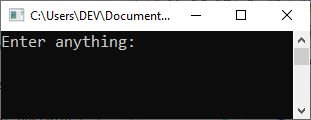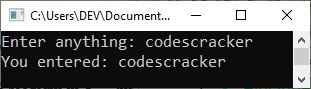# C++ Input and Output Operators

In this article, you will learn about the two most commonly used operators in C++, the input (>>) and the output (<<) operators. These two operators are used every time we need to receive input from the user and output the data to the output console. So without any further delay, let's start.

Input coming from the user's terminal, referred to as "standard input," is tied to the predefined iostream cin, and output directed to the user's terminal, referred to as "standard output," is tied to the predefined iostream cout.

## C++ Output Operator

The output operator ("<<") ("put to"), also called the stream insertion operator, is used to direct a value to standard output.

### C++ Output Operator Example

The following C++ program is an example demonstrating the output operator.

```#include<iostream>
using namespace std;
int main()
{
cout<<"Hello and thank you for visiting codescracker\n";

return 0;
}```

Here is the sample output of the above C++ program:Please note: The "\n" is used to insert a line break on the output console.

## C++ Input Operator

The input operator (">>") ("get from"), also known as the stream extraction operator, is used to read a value from standard input.

### C++ Input Operator Example

Following is an example program in C++ that uses the C++ input operator, which is ">>.".

```#include<iostream>
using namespace std;
int main()
{
int num;
cin>>num;

return 0;
}```

If you execute the above C++ program, you will get nothing in the output. The only thing you need to do with the output console is enter a number, say "23," and hit the "ENTER" key to see the following output:The number "23" is received and stored in the variable "num." But before ending the execution of the program, we did not utilize this value. Now let's create another example that uses both the input and output operators in a single program. That is, first I received the input from the user and then output the entered data to the output console.

```#include<iostream>
using namespace std;
int main()
{
char myvar;
cout<<"Enter anything: ";
cin>>myvar;
cout<<"You entered: "<<myvar;
cout<<endl;

return 0;
}```

The following snapshot shows the initial output produced by the above C++ program:Now supply any input, say "codescracker," and hit the enter key to see the following output:Since the variable "myvar" is defined with a maximum size of 20 characters, try to input anything not exceeding more than 20 characters.

The above example can also be written as:

```#include<iostream>
using namespace std;
int main()
{
char myvar;
cout<<"Enter anything: ";
cin.getline(myvar, 20);
cout<<"You entered: "<<myvar;
cout<<endl;

return 0;
}```

#### More Examples

Here are some more C++ example programs listed that you can go for:

C++ Quiz

« Previous Tutorial Next Tutorial »